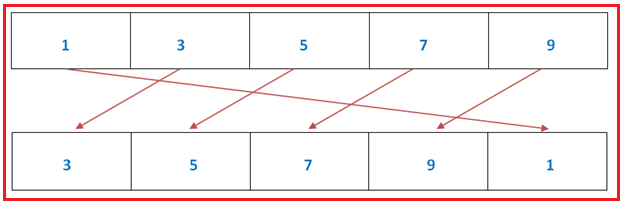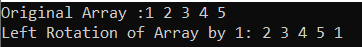# Left Rotation of Array by 1 in C#

## Left Rotation of Array by 1 in C# with Examples

In this article, I am going to discuss the Left Rotation of Array by 1 in C# with Examples. Rotation of an array basically means shifting each and every element to a specified position to the left or right. While writing the program for array rotation we should consider 2 factors:

1. The direction of Rotation – In what direction do we want to perform the rotation. Whether it would be left to right (clockwise) or right to left (anticlockwise).
2. Position of Rotation – At which position rotation will start.

1. Left Rotation of array by 1.
2. Right Rotation of array by 1.
3. Rotation of array by position k
4. Popup and Unshifting
5. Reversal Algorithm
6. Juggling Algorithm
##### Left Rotation of array by 1 in C#In this rotation of the array, each element will be shifted to the left side by position 1. This basically means the value of index 1 will be shifted to index 0, index 2 value will be shifted to index 1, and so on.

Example: From the above fig. We have an array with 5 elements.
Arr = 1,
Arr = 3,
Arr = 5,
Arr = 7,
Arr = 9

So, after performing the left rotation of array 1 the updated values of the array will be
Aar = 3,
Arr = 5,
Arr = 7,
Arr = 9,
Arr = 1

As from the above explanation, it is very clear to us that the changes will be as follows:
Arr = 1 => Aar = 3
Arr = 3 => Arr = 5
Arr = 5 => Arr = 7
Arr = 7 => Arr = 9
Arr = 9 => Arr = 1

Here, we are basically doing what is that except for the index 0 we just need to select the value of indexes one by one and shift that value to the previous index.

##### Algorithm to write code

Step1: Create a Temp variable to store the value at index 0.
Step2: Sift all the elements of array to left side by position 1 i.e. arr[i] = arr[i+1];
Step3: Store the value of temp at the last index of array i.e. arr[(arr.Length – 1)] = x;

##### C# Program to perform left rotation of array by position 1.
```using System;
public class LeftRotationOfArray
{
static void Main(string[] args)
{
int[] arr = new int[] { 1, 2, 3, 4, 5 };
Console.Write("Original Array :");
for (int i = 0; i < arr.Length; i++)
{
Console.Write(arr[i] + " ");
}

Console.WriteLine();

//Object declaration of class to access LeftRotate method
LeftRotationOfArray obj = new LeftRotationOfArray();

Console.Write("Left Rotation of Array by 1: ");
obj.LeftRotate(arr);
for (int i = 0; i < arr.Length; i++)
{
Console.Write(arr[i] + " ");
}

}

void LeftRotate(int[] arr)
{
int x = arr;
for (int i = 0; i < (arr.Length - 1); i++)
{
arr[i] = arr[i + 1];
}
arr[(arr.Length - 1)] = x;
}
}
```
###### Output:In the next article, I am going to discuss the Right Rotation of Array by 1 in C# with Examples. Here, in this article, I try to explain the Left Rotation of Array by 1 in C# with Examples and I hope you enjoy this Left Rotation of Array by 1 in C# article.